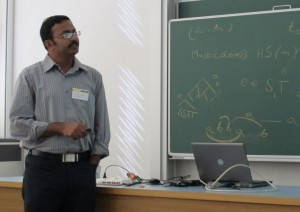# Sourav Chakraborty

## Property Testing: Sublinear Algorithms for Promise Problems

 DATE: Saturday, September 3, 2011ABSTRACT

Deciding weather a graph is k-colorable is an NP-complete problem and hence solving this problem is expected to be hard. But if we are given a promise that the graph is either k-colorable of "far from being k-coloarble", can we make some intelligent deductions "quickly"? Property testing deals with these kind of questions, where the goal is to solve some promise problems. The efficiency of an algorihtm is measured by the number of input bits that are read. In many cases there are algorithms that can correctly answer with high probability by looking at a tiny fraction (sometimes even constant) of the input bits. In the past two decades this area has been at the forefront of research in theoretical computer science - we will take at look at it.

Given at WorKer 2011 – The Third Workshop on Kernelization (slides are linked there).